## A particular moth can infest avocado trees and potentially damage the avocado fruit. A farmer plans to investigate the number of damaged avo

Question

A particular moth can infest avocado trees and potentially damage the avocado fruit. A farmer plans to investigate the number of damaged avocado fruit on moth-infested avocado trees of a certain variety. A recent article states that the population distribution of the number of damaged avocado fruit on moth-infested avocado trees of this variety is symmetric with mean of 6.4 and standard deviation of 1.9.

Required:

Compare the shapes of the sampling distributions of the sample mean number of damaged avocado fruit for random samples of 6 moth-infested trees and for random samples of 90 moth-infested trees from the population. Explain your answer.

in progress 0
2 months 2021-08-25T17:08:38+00:00 1 Answers 0 views 0

By the Central Limit Theorem, both distributions are normal, with mean of 6.4. For samples of size 6, the standard deviation of the the sampling distributions of the sample mean number of damaged avocado fruit is of 0.78, while for samples of 90, it is of 0.2.

Step-by-step explanation:

Central Limit Theorem

The Central Limit Theorem estabilishes that, for a normally distributed random variable X, with mean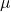and standard deviation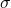, the sampling distribution of the sample means with size n can be approximated to a normal distribution with meanand standard deviation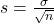.

For a skewed variable, the Central Limit Theorem can also be applied, as long as n is at least 30.

A recent article states that the population distribution of the number of damaged avocado fruit on moth-infested avocado trees of this variety is symmetric with mean of 6.4 and standard deviation of 1.9.

So normally distributed, with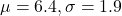Samples of 6:

This means that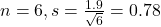The mean is the same.

Samples of 90:

This means that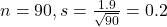The mean is the same.

By the Central Limit Theorem, both distributions are normal, with mean of 6.4. For samples of size 6, the standard deviation of the the sampling distributions of the sample mean number of damaged avocado fruit is of 0.78, while for samples of 90, it is of 0.2.